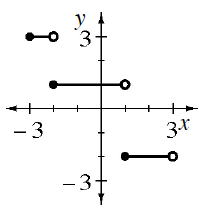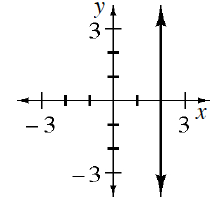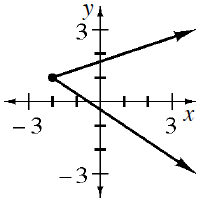### Home > INT3 > Chapter Ch1 > Lesson 1.2.2 > Problem1-98

1-98.

For each graph, what is the domain and range?

Domain: This set consists of every input value for $x$ for which the function is defined.
Range: the set of possible values of the output. This set contains every $y$-value that the function can generate.

1.Domain: $-3 ≤ x ≤ 3$
Range: $y = -2, 1, 3$

1.Domain: $x = 2$
Range: $-∞ < y < ∞$

1.